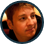Geoff Blair

# Rain Effect in HTML5 Canvas

April 13, 2016

While developing an outdoor environment for A Wizard's Lizard: Soul Thief, I wanted to create a rain effect to give the area a distinct feel.

Check out the video below to see the rain effect in action:

This effect is fairly simple to create so let's dive in to how it works. We're not going to cover every single line of code in this post, but you can check out the full source on GitHub.

The basic concept is to manage a collection of drops which have a few properties:

• A point in 2D space (x, y)
• Velocity
• Length
• Opacity

By varying the velocity, length, and opacity, we get drops that aren't completely uniform and feel a bit more natural. For each drop, these same properties are scaled together so that drops with higher velocity are also longer and less transparent which gives the illusion of depth.

## Initializing Drops

Let's take a look at how we initialize our drops:

``````// Collection of rain drops
var drops = [];

var initDrops = function () {
for (var i = 0; i < DROP_COUNT; i++) {
var drop = {};
resetDrop(drop);
drop.y = math.randomInteger(0, stage.height);
drops.push(drop);
}
};

// Reset a drop to the top of the canvas
var resetDrop = function (drop) {
var scale = Math.random();
drop.x = math.randomInteger(-DROP_X_BUFFER, stage.width + DROP_X_BUFFER);
drop.vx = WIND_VELOCITY;
drop.vy = math.lerp(DROP_MIN_VELOCITY, DROP_MAX_VELOCITY, scale);
drop.l = math.lerp(DROP_MIN_LENGTH, DROP_MAX_LENGTH, scale);
drop.a = math.lerp(DROP_MIN_ALPHA, DROP_MAX_ALPHA, scale);
drop.y = math.randomInteger(-drop.l, 0);
};``````

Notice that we create all the drops at once in `initDrops` and we have a separate function `resetDrop` which resets the state of a drop. This allows us to reuse the drop object once it reaches the bottom of the screen instead of destroying it and creating a new object.

During init, we also randomize the drop's Y value so that the drops are spread out along the Y axis from the start instead of always starting at the top when the effect begins.

We have a couple math helper functions here, too. `math.randomInteger` is pretty straight-forward and simply returns an integer between a min and max. We use this to randomize the placement of the drop along the X axis. `math.lerp` is a linear interpolation function and is used to scale a drop's velocity, length, and opacity by some normal value (between 0 and 1).

## Updating Drops

In order for the rain effect to animate we need to update the drops each frame. We use `requestAnimationFrame` to receive a callback to update and render our drops.

The `updateDrops` function is very simple. Loop over each drop and update its position based on velocity. If the drop is off the screen, reset it.

``````var updateDrops = function (dt) {
for (var i = drops.length - 1; i >= 0; --i) {
var drop = drops[i];
drop.x += drop.vx * dt;
drop.y += drop.vy * dt;

if (drop.y > stage.height + drop.l) {
resetDrop(drop);
}
}
};``````

## Rendering Drops

The final step is to render the drops to the canvas. We loop over the drops again and use the canvas line drawing APIs draw them.

``````var renderDrops = function (ctx) {
ctx.save();
ctx.strokeStyle = DROP_COLOR;
ctx.lineWidth = DROP_WIDTH;
ctx.compositeOperation = "lighter";

for (var i = 0; i < drops.length; ++i) {
var drop = drops[i];

var x1 = Math.round(drop.x);
var y1 = Math.round(drop.y);

var v = { x: drop.vx, y: drop.vy };
math.normalizeVector(v);
math.scaleVector(v, -drop.l);

var x2 = Math.round(x1 + v.x);
var y2 = Math.round(y1 + v.y);

ctx.globalAlpha = drop.a;
ctx.beginPath();
ctx.moveTo(x1, y1);
ctx.lineTo(x2, y2);
ctx.stroke();
ctx.closePath();
}
ctx.restore();
};``````

The only tricky thing going on here is the calculation of the drop's start end end points. We use a little bit of vector math to accomplish this by creating a vector which represents the drop's velocity then normalizing it and scaling it by the drop's length. A normalized vector (or unit vector) is a vector with magnitude of 1.

## Wrapping Up

Here's the final rain effect:

Remember to take a look at the full source on GitHub to fill in any gaps.

We didn't discuss splashes in this post, but they can be accomplished in a very similar manner and rendered using the `arc` canvas API.